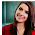# Computer System Architecture Set 22

## Questions 211 to 220

 211 ________bits are used to denote a character. (a)  16                             (b)  7                               (c)  2                               (d)  8                               (e)  32. 212 The fastest processor chips today have an internal clock rate of at least ______. (a)  100 MHZ               (b)  200 MHZ               (c)  400 MHZ                (d)  250 MHZ    (e)  300 MHZ. 213 Octal number 736.4 is converted to decimal as (a)  (475.5)10                           (b)  (479.5)10                           (c)  (478.5)10                           (d)  (476.3)10                           (e)  (477.5)10. 214 Conversion of the decimal 41.6875 into binary number will be (a)    110001.1101                                                (b)  10000.1011           (c)  101001.1011 (d)  101101.1011                                                 (e)  101111.1011. 215 The addition of two binary numbers in 2’s complement x=1010100 and y=1000011 (a)    11010110             (b)  11110011               (c)  01111011               (d)  10010001  (e)  11000111. 216 Floating point is always interpreted to represent a number in the following form (a)    M * re                               (b)  Me * r                      (c)  E r * m                     (d)  M * e                       (e)  Em * r. 217 If 1’s are mapped in a k-map the Boolean function is in the form (a)    Sum of products                                          (b)  Product of sums (c)    Subtraction and divide                               (d)  Addition and subtraction             (e)  Sum of sums. 218 A combinational circuit that performs the addition of two input bits and a carry from the previous lower significant position is called (a)  Full-adder                                                       (b)  Half-adder                         (c)  Full-subtractor                                (d)  Half-subtractor                                              (e)  Full-Divider. 219 Which of the following gates is called as a universal gate as any digital system can be implemented with it? (a)  AND gate                (b)  NAND gate             (c)  OR gate                   (d)  SR gate    (e)  XOR gate. 220 A combinational circuit that converts binary information from n input lines to a maximum of 2n unique output lines is (a)  Decoder                                                           (b)  Encoder                  (c)  Full adder (d)  Half adder                                                      (e)  Half-Subtractor.

1.